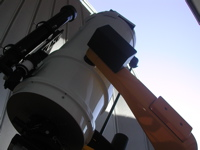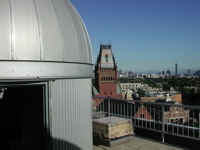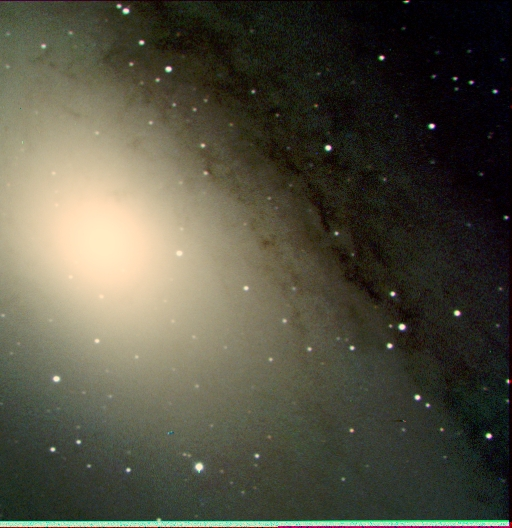# Astronomy Lab and Clay TelescopeCCD Observations of the Andromeda Galaxy - EL Obs 4

SPU-21 Fall 2014

printer version

In this lab, we will measure one of the most basic properties of a galaxy: its mass and stellar composition. Our goal is to measure the B, V, R magnitudes of the center Bulge (bright region around the nucleus) of the Andromeda galaxy (M31) and deduce its age. Using the integrated magnitude in V-band at several different aperture radii), we will deduce the approximate total stellar mass within each radius, given your measured B-V color and thus the spectral type and mass within that radius.

Observations:

• We must begin by observing our standard star for calibration.
• We will choose a bright star (V=4.52, B=4.39) that is only ~1 degree NW of M31 at J2000 coords: RA, DEC = 00:49:48.847, +41:04:44.08
• This star is ν-Andromeda, a B star with an F star companion (spectroscopic binary and not variable).
• Slew to this object and take 2 exposures of ~3seconds in each of the B, V, R filters. Enter in observations table (pdf or docx). In the images, also note the sky background counts in each filter (well removed from the star).
• Now let's take our Andromeda images!• Use TheSky program to slew to M31.
• Using the eyepiece, center on the bright core of the bulge of the galaxy. The light you are seeing left the galaxy 2 million years ago!
• Now take a test exposure of 10 seconds in B, V, and R to ensure that the center pixels are < 30,000 counts/pixel and that out to 1 arcmin radius from the center there are still > 2,000 counts/pixel above the sky background (use the sky background, scaled to exp. time used for Andromeda).
• Once you have the appropriate exposure times, take 2 exposures in each filter and again enter the observations in your observations table.
• If you have extra time, move the telescope so you are slightly off-center from the bulge and re-take you B, V, and R image. You can then make a really nice "true" color image (to the right) of the central disk region of Andromeda - not just the Bulge!

Reductions:

• First we must reduce the calibration star, ν-Andromeda manually to derive the "const" in Equation 1 below, where S is the total star counts, B is the total background counts and T is the exposure time. Use the given B and V magnitudes give above.
• Apparent mag (B,V) = -2.5log[(S-B)/T] + const
• Enter these values in the reductions table (pdf or docx).
• Now we will reduce the Andromeda images. For each image follow the outlined steps.
• Using the above apparent magnitude equation (above), we will solve for the B and V magnitudes for M31, where S is the total counts inside the aperture radii (do this for radii 10 and radii 20), T is the exposure time, and B is the scaled background counts that you measure from your calibration. Enter these values in the above reductions table.

Analysis:

Now that you have your B and V magnitudes for M31 we can do some analysis. Use the HR Diagram Handout for reference. Enter your data into the analysis table (pdf or docx).

• Derive the (B-V) colors and thus approximate spectral types for each radius.
• Use your B-V value to estimate the age if these colors were the turnoff colors.
• What is the TOTAL mass (in solar masses) contained within the 2 apertures?
• Estimate this by using the distance to M31(d=700kpc) in the distance modulus equation, m-M=5log(d)-5, to get the absolute magnitude, MV M31, for each radius.
• Then convert to the equivalent number of Sun-like stars each with absolute magnitude of MV Sun, N = -100.4(MV M31 - MV Sun). Since the Bulge stars are not like the Sun, you would need to multiply this number of Sun-like stars, N, by the M/L ratio for the Bulge stars, but this (factor of ~2) correction can be ignored here.
• Derive the Mass Density of stars contained in each aperture radius.
• For the two aperture radii, derive the mass density in stars contained within each, ρ(<R) = Mass(<R)/Volume(<R).
• How do the two values compare and what does this imply for the radial distribution of stars in the Bulge - is it consistent (roughly) or falling off with increasing radius R?
• Finally (optional if there is time!), make a color (BVR) image of M31.
• Follow the steps for Making a Color Image.
• Unfortunately, our image is not wide or deep enough to show us the spiral arms and disk of M31, but do you see any evidence for structure in the brightness of the Bulge or is it relatively uniform and smooth (similar to a globular cluster)?